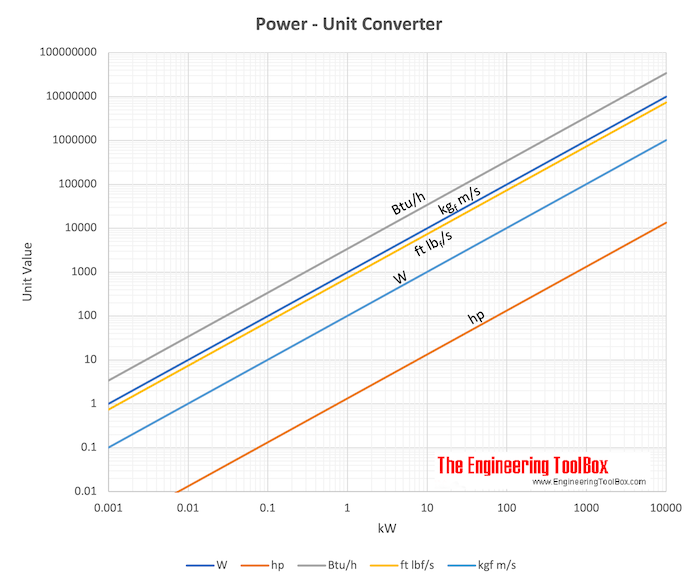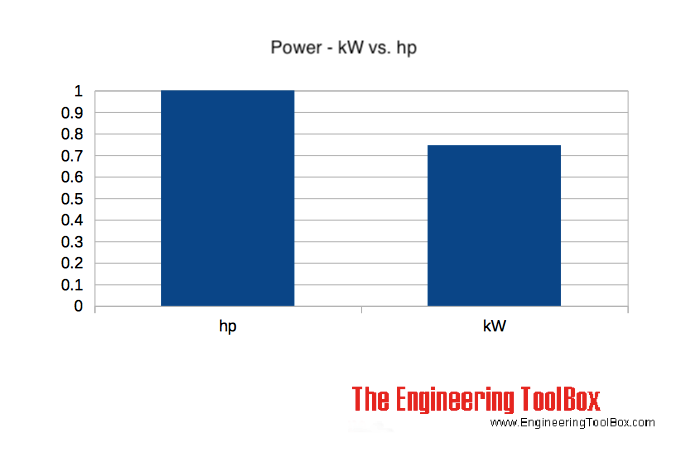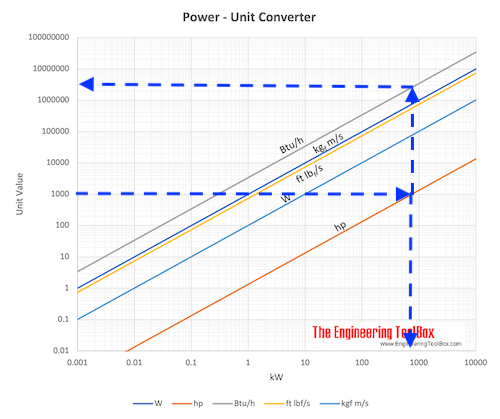Engineering ToolBox - Resources, Tools and Basic Information for Engineering and Design of Technical Applications!

# Power

## Power is the rate at which work is done or energy converted.

### Work done

Power is a measure of the rate at which work is done - work done per unit time - and can be expressed as

P = Work / time

= W / dt                                (1)

where

P = power (W, ft lb/s)

W = work done (J, ft lb)

dt = time taken (s)

Work can be expressed as

W = F s (1b)

where

F = force (N, lb)

s = distance (m, ft)

Since work is the product of the applied force and the distance, power (1) can be modified to

P = F v                               (1c)

where

F = force (N, ft)

v = velocity (m/s, ft/s)

### Energy converted

Power is also a measure of the rate at which energy is converted from one form to another and can be expressed as

P = E / dt                            (2)

where

P = power (W, ft lb/s)

E = energy converted (J, ft lb)

dt = time taken (s)

### Example - Power needed to lift a Mass

A mass of 1000 kg is lifted 10 m in 10 seconds.

The work done can be calculated as

W = (1000 kg) (9.81 m/s2) (10 m)

= 98100 J (Nm)

The power required is work done per unit time

P = (98100 J) / (10 s)

= 9810 W (J/s)

= 9.8 kW

### Example - Work done by an Electric Motor

The work done by a 1 kW electric motor in 1 hour can be calculated by modifying (1) to

W = P dt

= (1 kW) (1000 W/kW) (1 h) (3600 s/h)

= 3600000 J

= 3600 kJ

### Example - Electric Winch lifting a Mass

An electric winch is lifting a mass of 100 kg 10m above the ground. The electric motor in the winch is 500 W. The force (weight) acting on the mass due to the acceleration of gravity can be calculated as

F = m g

= (100 kg) (9.81 m/s2)

= 981 N

= 0.98 kN

The work done by the winch can be calculated with (1b) as

W = F s

= (981 N) (10 m)

= 9810 Nm (J)

The time required for the winch with the actual motor to lift the mass can be calculated by modifying (1)

dt = W / P

= (9810 J) / (500 W)

= 19.6 s

### Power - kW versus HorsepowerPower can also be expressed in horsepower (hp):

1 kW = 1.341 hp

1 hp = 0.7457 kW• 1 kW = 1000 W = 3412 Btu/h = 737.6 ft lbf/s = 1.3596 hp(M) (ps) = 1.341 hp(I) (bhp) = 101.97 kgf m/s = 737.6 ft lbf/s
• 1 hp (English horse power, bhp) = 745.7 W = 0.746 kW = 550 ft lb/s = 2545 Btu/h = 33000 ft lb/m = 1.0139 ps (metric horse power) ~= 1.0 KVA

PS (Pferdestärke, horse strength in German) referred to as 'metric horsepower' is replaced by kW as the legal EU measurement of engine power.

### Comparative Power Outputs

Comparative power outputs are indicated below:

• human sound - normal speech : 10-8 kW
• human output: 0.1 kW (daily average), 0.2 kW (walking), 1 kW (running), 1.7 kW (sprinting)
• small scooter : 4 kW
• family car : 40 kW
• light aircraft : 150 kW
• sports car : 240 kW
• helicopter : 400 kW
• formula 1 car : 600 kW
• locomotive : 1500 kW
• cargo vessel : 6000 kW
• military aircraft : 30000 kW
• warship : 60000 kW
• airliner : 80000 kW
• launch rocket : 400000 kW

### Example - 1000 hp

1000 hp can be converted to aprox. 745 kW or 2500000 Btu/h as indicated in the chart below.## Related Topics

• ### Mechanics

Forces, acceleration, displacement, vectors, motion, momentum, energy of objects and more.

## Related Documents

• ### Angular Motion - Power and Torque

Angular velocity and acceleration vs. power and torque.
• ### BFD - Block Flow Diagram - Example

Example Block Flow Diagram - BFD.
• ### Efficiency

Efficiency is the ratio useful energy output to energy input.
• ### Electrical Equipment - Power Consumption

Typical power consumption and running time for common electrical equipment.
• ### Electrical Motors - Shaft Power vs. Voltage and Current

Calculate electric motors shaft power.
• ### Elevators - Force and Power

Required force and power to lift an elevator.
• ### Energy

Energy is the capacity to do work.
• ### Fans - Efficiency and Power Consumption

Power consumption and typical efficiencies of fans
• ### Power Gained by Fluid from Pump or Fan

Calculate the power gained by fluid from an operating pump or fan.
• ### Pump Power Calculator

Calculate pumps hydraulic and shaft power.
• ### Pumping Water - Required Horsepower

Horsepower required to pump water.
• ### Pumps, Fans and Turbines - Horsepower

British Horse Power as used for pumps, fans and turbines - and how to convert to other units.
• ### Single Phase Power Equations

Power equations for single phased electrical systems.
• ### Three-Phase Electrtical Motors - Power Factor vs. Inductive Load

Inductive loads and power factors with electrical three-phase motors.
• ### Three-Phase Power - Equations

Electrical 3-phase equations.
• ### Torque - Work done and Power Transmitted

The work done and power transmitted by a constant torque.
• ### Winches

Effort force to raise a load.
• ### Wind Power

Power generation from wind.
• ### Wind Turbine Power Calculator

Online wind turbine calculator.
• ### Work done by Force

Work done by a force acting on an object.

## Engineering ToolBox - SketchUp Extension - Online 3D modeling!

Add standard and customized parametric components - like flange beams, lumbers, piping, stairs and more - to your Sketchup model with the Engineering ToolBox - SketchUp Extension - enabled for use with older versions of the amazing SketchUp Make and the newer "up to date" SketchUp Pro . Add the Engineering ToolBox extension to your SketchUp Make/Pro from the Extension Warehouse !

We don't collect information from our users. More about

## Citation

• The Engineering ToolBox (2008). Power. [online] Available at: https://www.engineeringtoolbox.com/power-d_1289.html [Accessed Day Month Year].

Modify the access date according your visit.

9.29.12